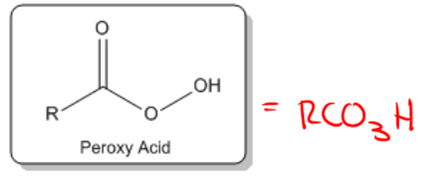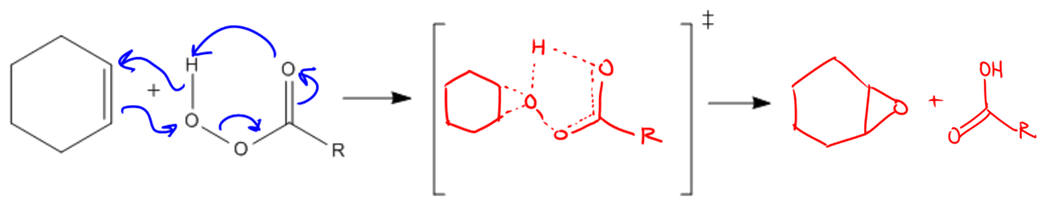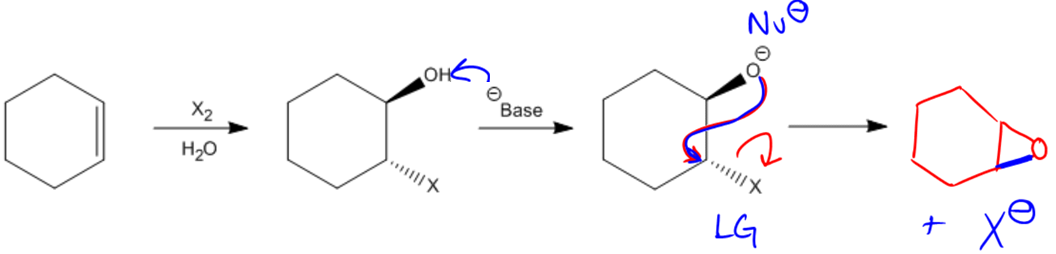## Organic Chemistry

Learn the toughest concepts covered in Organic Chemistry with step-by-step video tutorials and practice problems by world-class tutors.

# Epoxidation

This reaction adds a 3-membered cyclic ether (epoxide functional group) to an alkene using reagents called peroxy acids. These epoxides are highly strained, so they can react in very useful ring-opening reactions, which we will discuss later.

Epoxides from Peroxy Acids
1
concept

## General properties of epoxidation.2m
Play a video:
So now I want to talk about another addition. Reaction. And this one on Lee adds oxygen by itself toe a double bond to form a completely new functional group called in a pox side. Needless to say, the name of this reaction is called hip oxidation. So before we can even get started, I kind of wanted to find what is in a pox side because some of you guys might not know, all right. And in a pox, it is a functional group that's just made of a cyclic three member either. So what that means is that remember that the definition of an ether was R O R. That was an ether. Okay, well, in a pox side is just gonna be a cyclic ether. So what that means is it's an o, uh, with two are groups on both sides, but they're attached to each other, so this would be in a park side. Okay. Approximation, Needless to say, is gonna add that one oxygen to the double bond in order to make it into the three member ring. Okay, so how do we add a pox sides? Two double bonds. What? We do it by using a type of molecule called a proxy acid. Okay, proxy acids are the molecules that are used to make them. And this is the general formula of a proxy acid. What you're going to notice is that it actually looks a lot like a car box. Cilic acid. Remember that Carve oxalic acid. Oops. Remember that car looks like acid. Looks like this. Ohh. So, really, it's the same thing as a car Oxalic acid. Except as one more. Oh, so remember that the definition of a car oxalic acid is C O to h. That's the condensed formula. Well, for a proxy acid, it's gonna be our C h. So what you're going to notice is that it's really the same exact thing. Except I just added one more oxygen. So its CEO three h all right, so that's the first thing. Okay, Now you could use any proxy acid. You want to make it a pox side, but the common ones that air used R M c P, B A and M MPP these air to re agents that you don't need to know exactly what they look like as long as you can recognize that these are types of proxy acids. Okay, The only thing that changes is the our group. But the C O. H. Is the same. All right?

Peroxy acids are compounds with the general molecular formula RCO3H. The most common examples are MCPBA and MMPP. These are essentially the same molecule, just with different –R groups.2
concept

## The mechanism of how peroxy acids make epoxides.3m
Play a video:
so the mechanism for this guy is actually kind of crazy. Ah, lot professors don't need you to know it, but some do. So I'm just gonna teach to anyway, just in case. Also, because I want you guys to be the smartest kids in the class, so might as well learn it. Okay, so where do you think the first arrow is gonna come from? That part is easy. The first arrow comes from the double bond. That's why nuclear file. And it turns out that it's going to attack the very last Oh, on my proxy acid. So that part is easy. Now, everything else is a lot of arrows, because when I make that bond, I have to break a bond because that oxygen would break its octet if I just added a new bond to it. So what's gonna happen is that the electrons from this bond are gonna make a double bond here. Does that make sense? So far. So basically, if I make up on, I have to break upon. But now if I make a double bond here, that means that this carbon this carbon carbon would break its octet. So if I break that. If I make that bond, then I have to break this bond and move those electrons up to the O. Okay, But now that I have these electrons up to the oh, this always gonna have a negative charge, that negative charge is gonna be attracted to the H on the other side. So then the electrons from this oh are going to grab that H. Okay, But does h like to have two bonds? No, it doesn't. So what that means is that the electrons from here are finally gonna go back and attack the double bond. So you're gonna have five arrows in total. I know that explanation kind of sounded crazy. That's probably the best way that we can think about it. Think about how the dull bonus starting it, and then there's kind of just like a flow of transfer of electrons through the whole molecule. Now, what you can imagine is that this is gonna make a crazy looking transition state. So, like I said, 90% of professors are not going to require you to draw this. But just in case you might have that one guy that needs you to do it. So we're gonna go ahead and do it, so we're gonna have to draw everything small because there's a lot of pieces here. What the transition state is gonna look like is that all these bonds that are being created and destroyed have to be drawn is partial bonds. So it's gonna be partial partial than a partial bond toe. Oh, then a partial, the straight A single bond, But then a partial double bond than a single bond to oh, single bonds are with a partial double bond. Then this has a partial bond to H, and then this has a partial bond to this. Oh, okay. Isn't that crazy? So that is what the transition state is gonna look like. Basically, partial bonds in every single reaction, every single place that we were making or breaking a bond is gonna be a partial bond at the end. What we're just going to get is that we're going to get in the Parkside, which just looks like this. Oh, with two bonds and then on the other side, we wind up actually getting a carb oxalic acid. Okay, so I mean, I kind of drew that wrong. It would be, um, in o h up here and then a double bond. Oh, here and then in our, which is a carb oxalic acid. Okay, so those are the two products, the one we really care about is the A pox side. Okay, because that's what we're gonna use in the following steps. Okay, So this is the number one way to make a pox sides. You're going to see this over and over again in future chapters, even you're going to see this.

You typically won’t need to know this entire mechanism, but here is the first step:

General Reaction:Note: There should also be a partial bond drawn in where the double bond used to be on the cyclohexane.

Epoxides from Halohydrins
3
concept

## Halohydrins to epoxides via intramolecular SN2.2m
Play a video: